# RD Sharma Solutions Class 12 The Plane Exercise 29.8

RD Sharma Solutions for Class 12 Maths Chapter 29 The Plane Exercise 29.8, is provided here for students to grasp the concepts easily and score well in the exams. The subject experts have framed and solved the questions accurately from every section. The RD Sharma Solutions are completely based on the exam-oriented approach to help students score well in their board examination. Students can easily download the pdf consisting of this chapter solutions, which are available in the links provided below.

## Download PDF of RD Sharma Solutions For Class 12 Maths Chapter 29 Exercise 8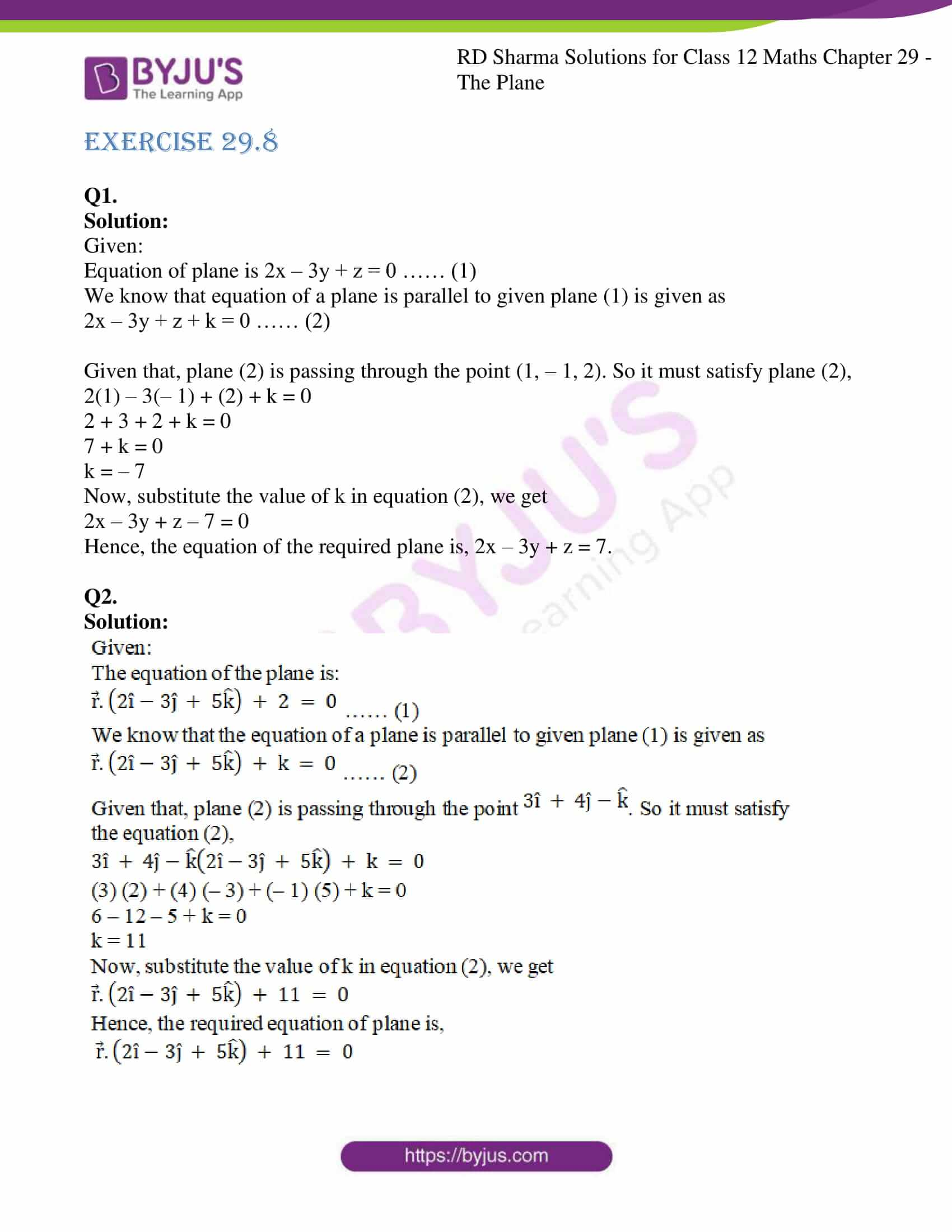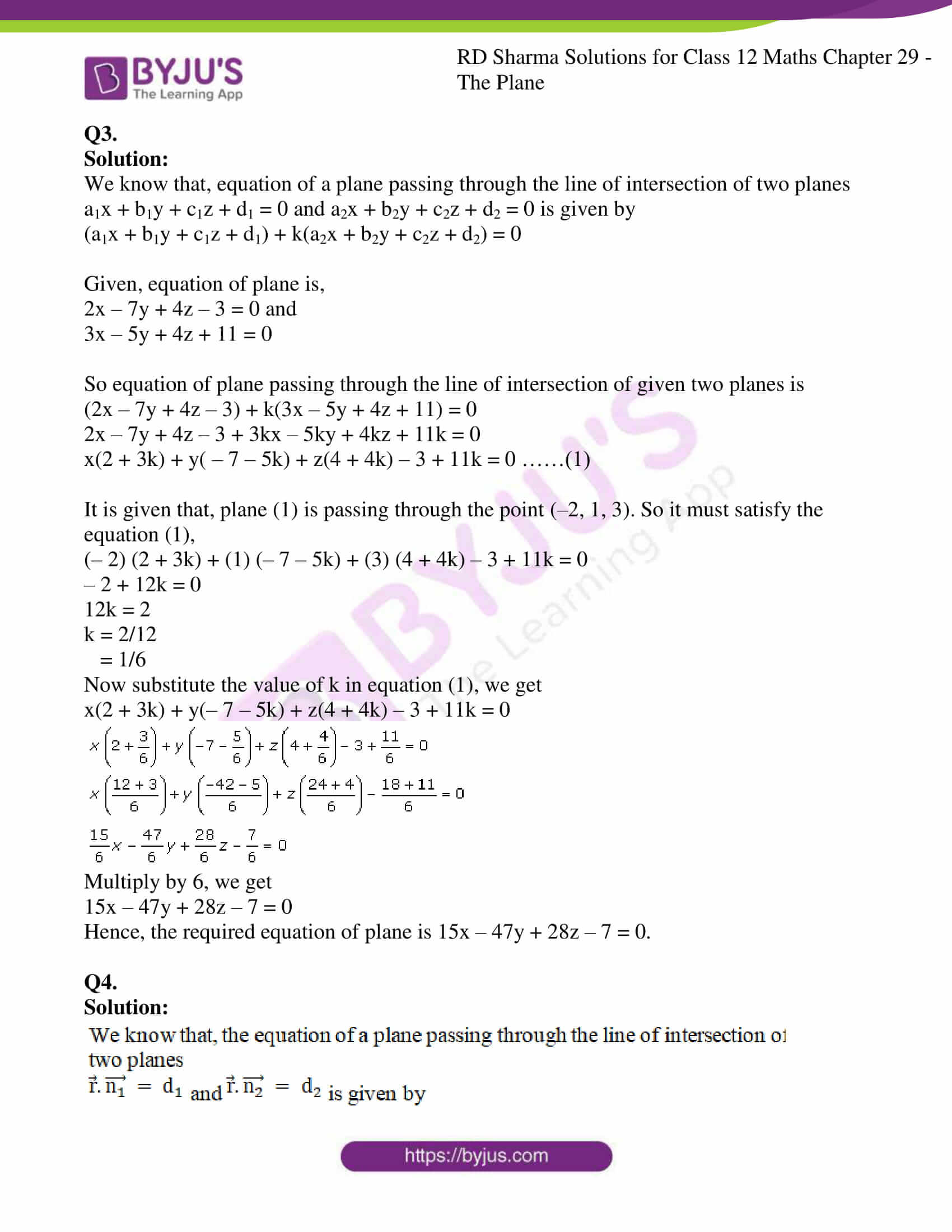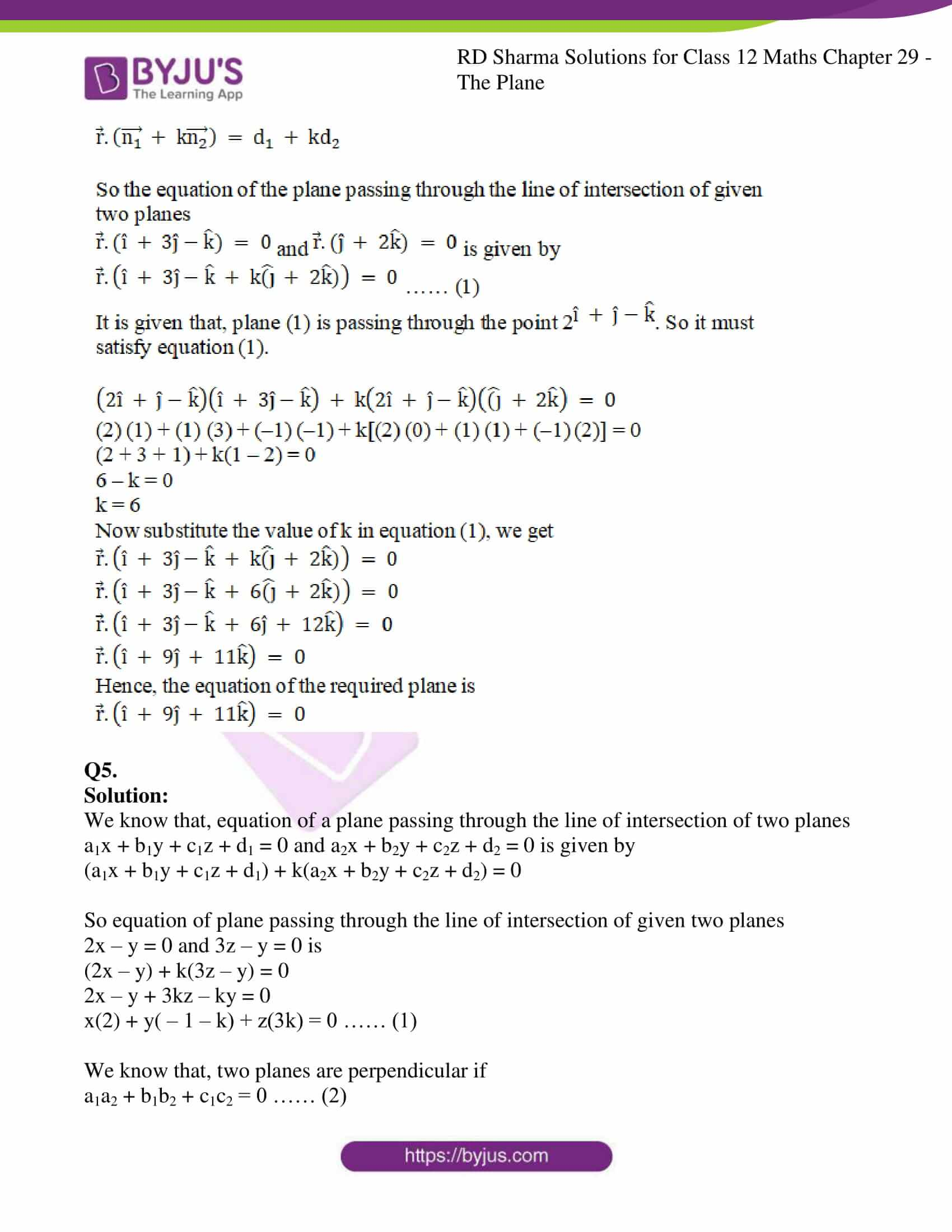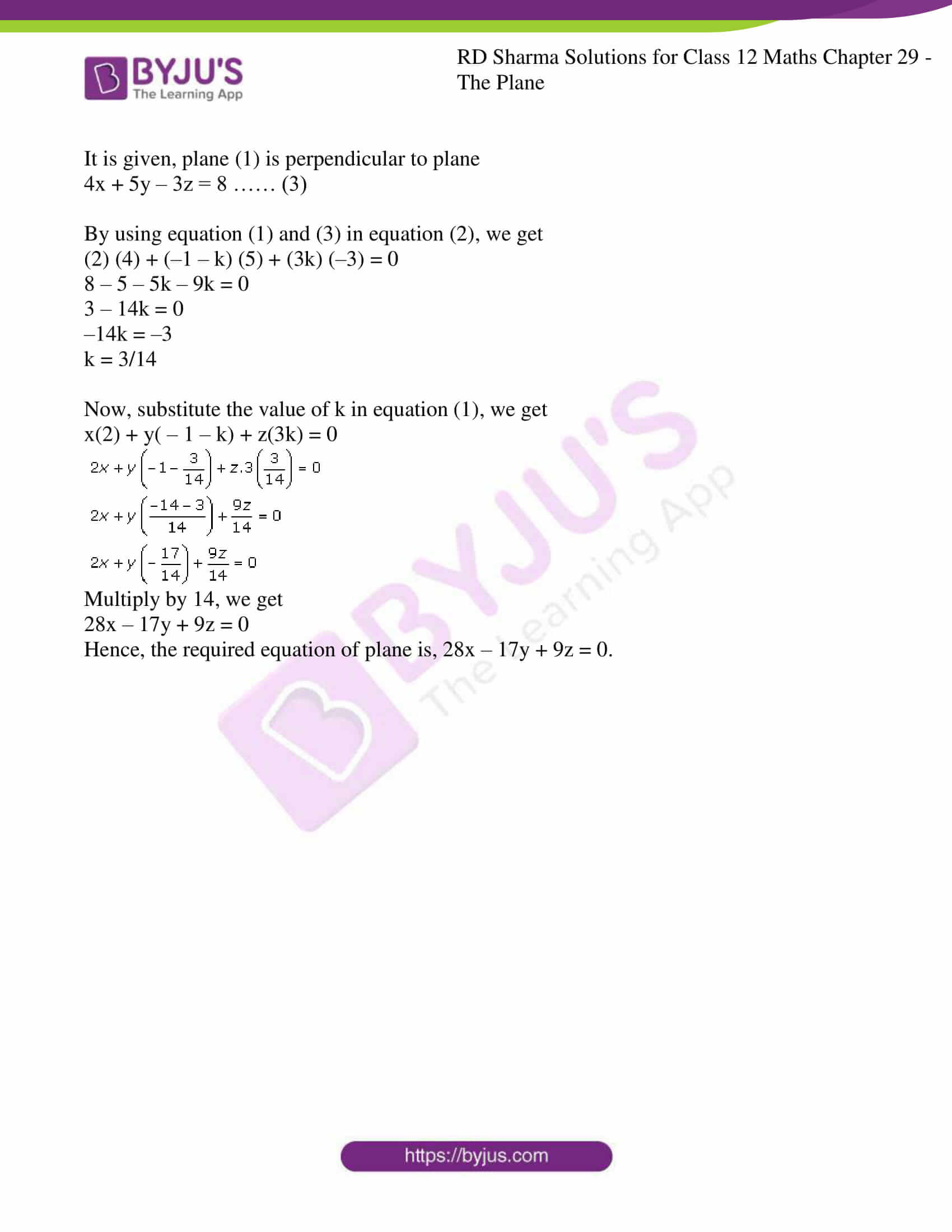### Access RD Sharma Solutions For Class 12 Maths Chapter 29 Exercise 8

EXERCISE 29.8

Q1.

Solution:

Given:

Equation of plane is 2x – 3y + z = 0 …… (1)

We know that equation of a plane is parallel to given plane (1) is given as

2x – 3y + z + k = 0 …… (2)

Given that, plane (2) is passing through the point (1, – 1, 2). So it must satisfy plane (2),

2(1) – 3(– 1) + (2) + k = 0

2 + 3 + 2 + k = 0

7 + k = 0

k = – 7

Now, substitute the value of k in equation (2), we get

2x – 3y + z – 7 = 0

Hence, the equation of the required plane is, 2x – 3y + z = 7.

Q2.

Solution: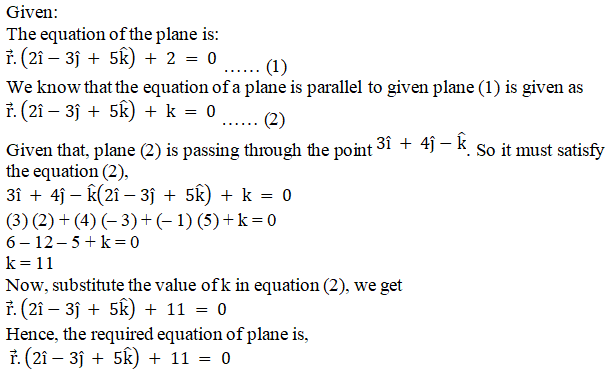Q3.

Solution:

We know that, equation of a plane passing through the line of intersection of two planes

a1x + b1y + c1z + d1 = 0 and a2x + b2y + c2z + d2 = 0 is given by

(a1x + b1y + c1z + d1) + k(a2x + b2y + c2z + d2) = 0

Given, equation of plane is,

2x – 7y + 4z – 3 = 0 and

3x – 5y + 4z + 11 = 0

So equation of plane passing through the line of intersection of given two planes is

(2x – 7y + 4z – 3) + k(3x – 5y + 4z + 11) = 0

2x – 7y + 4z – 3 + 3kx – 5ky + 4kz + 11k = 0

x(2 + 3k) + y( – 7 – 5k) + z(4 + 4k) – 3 + 11k = 0 ……(1)

It is given that, plane (1) is passing through the point (–2, 1, 3). So it must satisfy the equation (1),

(– 2) (2 + 3k) + (1) (– 7 – 5k) + (3) (4 + 4k) – 3 + 11k = 0

– 2 + 12k = 0

12k = 2

k = 2/12

= 1/6

Now substitute the value of k in equation (1), we get

x(2 + 3k) + y(– 7 – 5k) + z(4 + 4k) – 3 + 11k = 0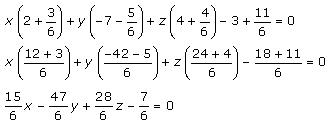Multiply by 6, we get

15x – 47y + 28z – 7 = 0

Hence, the required equation of plane is 15x – 47y + 28z – 7 = 0.

Q4.

Solution: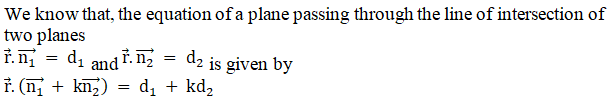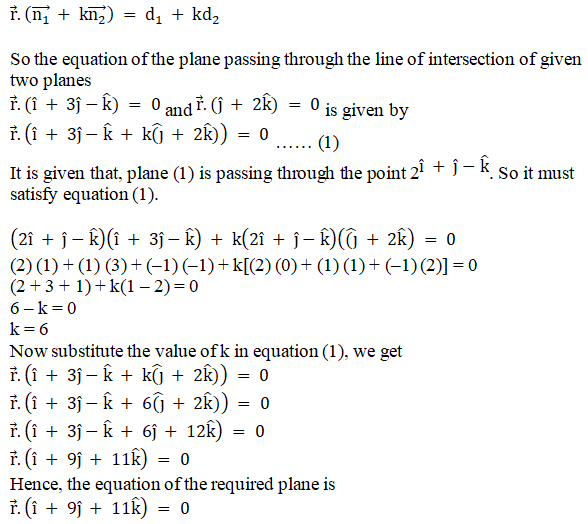Q5.

Solution:

We know that, equation of a plane passing through the line of intersection of two planes

a1x + b1y + c1z + d1 = 0 and a2x + b2y + c2z + d2 = 0 is given by

(a1x + b1y + c1z + d1) + k(a2x + b2y + c2z + d2) = 0

So equation of plane passing through the line of intersection of given two planes

2x – y = 0 and 3z – y = 0 is

(2x – y) + k(3z – y) = 0

2x – y + 3kz – ky = 0

x(2) + y( – 1 – k) + z(3k) = 0 …… (1)

We know that, two planes are perpendicular if

a1a2 + b1b2 + c1c2 = 0 …… (2)

It is given, plane (1) is perpendicular to plane

4x + 5y – 3z = 8 …… (3)

By using equation (1) and (3) in equation (2), we get

(2) (4) + (–1 – k) (5) + (3k) (–3) = 0

8 – 5 – 5k – 9k = 0

3 – 14k = 0

–14k = –3

k = 3/14

Now, substitute the value of k in equation (1), we get

x(2) + y( – 1 – k) + z(3k) = 0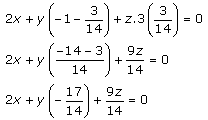Multiply by 14, we get

28x – 17y + 9z = 0

Hence, the required equation of plane is, 28x – 17y + 9z = 0.